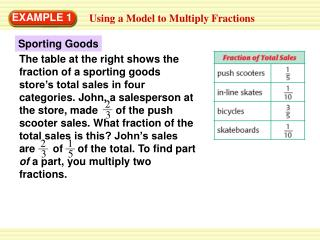Download PresentationEXAMPLE 1# EXAMPLE 1 - PowerPoint PPT Presentation

Download Presentation##### EXAMPLE 1

Download Policy: Content on the Website is provided to you AS IS for your information and personal use and may not be sold / licensed / shared on other websites without getting consent from its author. While downloading, if for some reason you are not able to download a presentation, the publisher may have deleted the file from their server.

- - - - - - - - - - - - - - - - - - - - - - - - - - - E N D - - - - - - - - - - - - - - - - - - - - - - - - - - -
##### Presentation Transcript

1. 2 2 1 3 3 5 EXAMPLE 1 Using a Model to Multiply Fractions Sporting Goods The table at the right shows the fraction of a sporting goods store’s total sales in four categories. John, a salesperson at the store, made of the push scooter sales. What fraction of the total sales is this? John’s sales are of of the total. To find part of a part, you multiply two fractions.

2. 1 1 15 15 1 2 1 1 2 1 2 2 3 5 3 5 3 5 5 3 2 = 15 2 15 EXAMPLE 1 Using a Model to Multiply Fractions To answer the question above about John’s sales, you can use a model to find , or . STEP 1Draw a 3 by 5 rectangle to model thirds and fifths. Each small square is of the whole. STEP 2Shade of the rectangle. STEP 3Select of the shaded rectangle. Two of the 15 squares are selected, so . John’s push scooter sales are of the store’s total sales. of ANSWER

3. 2 5 1 = = 7 5 5 3 1 2 2 3 4 15 = 28 1 4 15 10 EXAMPLE 1 Guided Practice for Example 1 Draw a model to find the product. 1. 2. 3.

4. EXAMPLE 2 Multiplying Two Fractions 2 5 2 5 = Use the rule for multiplying fractions. Multiply. Simplify. 3 5 3 5 10 = 15 2 = 3

5. 2 2 Substitute for n. 7 7 EXAMPLE 3 Evaluating an Algebraic Expression 2 1 Evaluate the expression when = . n n 7 3 1 1 n = 3 3 1 2 = Use the rule for multiplying fractions. 3 7 2 = Multiply. The product is in simplest form. 21

6. 1 1 5 2 6 3 5 3 5. Find the product 1 = 18 3 3 4 n 6. Evaluate when n= . 9 5 4 1 3 4 7 7. Evaluate when x= . 9 8 3 = = = 2 x 20 27 28 No. The product is less than but greater than . 3 for Example 2 and 3 GUIDED PRACTICE 4. Find the product 8.Is the product in Example 2 less than both fractions? Why or why not?

7. EXAMPLE 4 Simplifying Before Multiplying Football Kyle successfully completed 8 out of his 15 passes during one football season. Kyle’s friend Lou had a completion rate that was Kyle’s. What fraction of his passes did Lou complete during the season? 1 4 SOLUTION 1 8 1 8 = Use the rule for multiplying fractions. 4 15 15 4 2 1 8 = 4 is a factor of 4 and 8. Divide 4 and 8 by 4. 4 15 1

8. EXAMPLE 4 Simplifying Before Multiplying 1 2 = Rewrite. 1 15 2 = Multiply. 15 ANSWER 2 Lou completed of his passes. 15

9. 1 2 1 3 3 2 = 5 6 4 6 5 4 1 1 1 3 2 = 5 6 4 2 2 1 1 1 = 5 2 2 1 = 20 EXAMPLE 5 Multiplying Three Fractions Use the rule for multiplying fractions. 3 is a factor of 3 and 6. Divide 3 and 6 by 3. 2 is a factor of 2 and 4. Divide 2 and 4 by 2. Rewrite. Multiply.

10. 5 3 5 9. = 24 8 9 5 8 10. 16 15 1 3 7 2 1 1 3 = 11. 20 6 5 7 5 12. 1 1 10 = = 9 6 6 for Example4 and 5 GUIDED PRACTICE Find the product. Write the answer in simplest form.

11. c e a d f b ANSWER (a · c · e) (b · d · f) for Example4 and 5 GUIDED PRACTICE Look at the rule for multiplying fractions on page 349. Use variables to write a rule for multiplying the fractions , , and . 13.